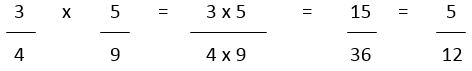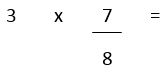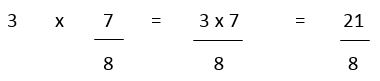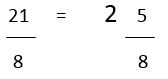# Multiplying Fractions and Whole Numbers

## Multiplying Fractions

Fractions often worry people and when you think about carrying out multiplication, the reaction from children is often one of real concern. However, there is nothing to fear, it is one of the most deceptively easy mathematical operations!

The natural thing for a child to do when they have been shown nothing of the theory, is to multiply the top numbers together and do the same with the bottom numbers. Luckily, that's all you have to do. The joy on children's faces when they hear this is great to watch!

Let's check out an example:The numbers are dealt with as if they were ordinary multiplication sums. The numerators (top numbers) are multiplied (3 x 5) and the denominators are multiplied (4 x 9). The answer then needs to be SIMPLIFIED. This is dealt with on another walkthrough but the basic idea is to find which number fits into both numerator and denominator and show how many times it does so.

One thing that appears counter-intuitive at first is that the answer will be less than the numbers you started with. When you multiply, you find 'x' lots of 'y', so ½ x ½ is the same as 'half lots of a half' and of course, that's less than a half! You should be wary of answers which are top-heavy / improper fractions after multiplying two normal fractions - the answer can't be correct.

## Multiplying Fractions and Whole Numbers

Sometimes you will face a whole number and a fraction which have to be multiplied together. This is not quite as straightforward as two fractions or two whole numbers but isn't far off. Look at the example below:

What is the answer when you multiply three by seven eighths?

Firstly, set it out like this:Now we can treat the sum like any other. Forget about needing a second denominator (when multiplying fractions we were multiplying two denominators together) as the denominator that is already there will do.We have simply multiplied the numerators together and left the single denominator on the bottom.

However, the answer is incomplete. We are left with a top-heavy or improper fraction, which has a numerator higher than the denominator. Without going over old ground too much, improper fractions can be changed into mixed numbers by finding how many times the denominator fits into the numerator. Therefore in our example, twenty-one eighths is the same as two whole ones and five eighths. The expectation would be that the answer is expressed as a mixed number so ensure your child doesn't think it is okay to leave the improper fraction.